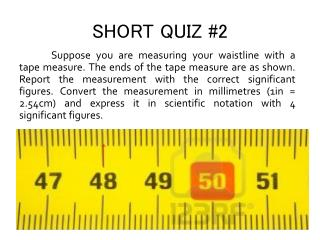DownloadDownload PresentationSHORT QUIZ #2

# SHORT QUIZ #2

Télécharger la présentation## SHORT QUIZ #2

- - - - - - - - - - - - - - - - - - - - - - - - - - - E N D - - - - - - - - - - - - - - - - - - - - - - - - - - -
##### Presentation Transcript

1. SHORT QUIZ #2 Suppose you are measuring your waistline with a tape measure. The ends of the tape measure are as shown. Report the measurement with the correct significant figures. Convert the measurement in millimetres (1in = 2.54cm) and express it in scientific notation with 4 significant figures.

2. One-dimensional motion Lecture #3 June 20 2013

3. Physical assumptions Every theory has a set of assumptions which specify the scope of its validity. One must be careful to know all the assumptions made to arrive at a particular conclusion

4. Relativity Speed is close to speed of light. Length scales are very large.

5. Quantum mechanics Mass and length scales are small Zinc atom on a layer of copper oxide

6. Classical physics • speed of the object << the speed of light • mass and length scales are comparable to “everyday objects”. • Ex: Air resistance and Coriolis force are neglected in projectile motion

7. Mathematical models Mathematical models are used to idealize the physical system and simplify the calculations. The accuracy of the solution is dependent on the faithfulness of the mathematical model to physical reality.

8. Free fall and projectile motion Projectile as point object: neglect rotational motion and microscopic fluctuations

9. Reference frames In solving motion problems, it is important to specify the reference frame. For most of our purposes, the reference frame is one or two dimensional and at rest.

10. Motion The only thing that constant in this world is change. All particles comprising the universe are in constant motion.

11. Two states of (translational) motion • Motion with constant velocity • Accelerated motion

12. Kinematics: Study of how objects move • Position • Distance and displacement • (Instantaneous and Average) Speed and velocity • (Instantaneous and Average) Acceleration

13. Position Position specifies where the object is in space.

14. Distance versus displacement Distance is the length of the trajectory of an object. Displacement is the change in position of the object with respect to the origin.

15. Speed versus velocity: Average Average speed is the change in distance divided by change in time. Average velocity is the change in displacement divided by change in time.

16. Speed versus velocity: Instantaneous Instantaneous speed Instantaneous velocity

17. Average and instantaneous acceleration Average acceleration is the change in velocity divided by change in time. Instantaneous acceleration:

18. Speeding up or slowing down?

19. Equations of motion with constant acceleration • Position: • Velocity: • Acceleration:

20. Free fall

21. Free fall motion assumptions: • The height is much smaller than the earth’s radius so that the acceleration is constant. • The apple is a point object; the motion is purely translational. • Only gravity influences the motion. Air resistance and other effects are neglected.

22. Free fall motion Given any two information, say, an initial velocity and position, the whole kinematics of free fall motion can be worked out. Why two?

23. Interpreting motion plots

24. Projectile Motion isconstantly accelerated vertical motion + motion with constant horizontal velocity

25. Horizontal and vertical motion are independent.

26. THREE POINTS: • In the analysis of the motion of an object, one must know the underlying physical assumptions, use idealized models, and specify the reference frame. • The kinematics of an object can be described by the following scalar or vector quantities: position, distance, displacement, as well as, average and instantaneous speed, velocity and acceleration. • Free fall is the one-dimensional motion of objects under the influence of the Earth’s gravity only. If in two dimensions, we have projectile motion.